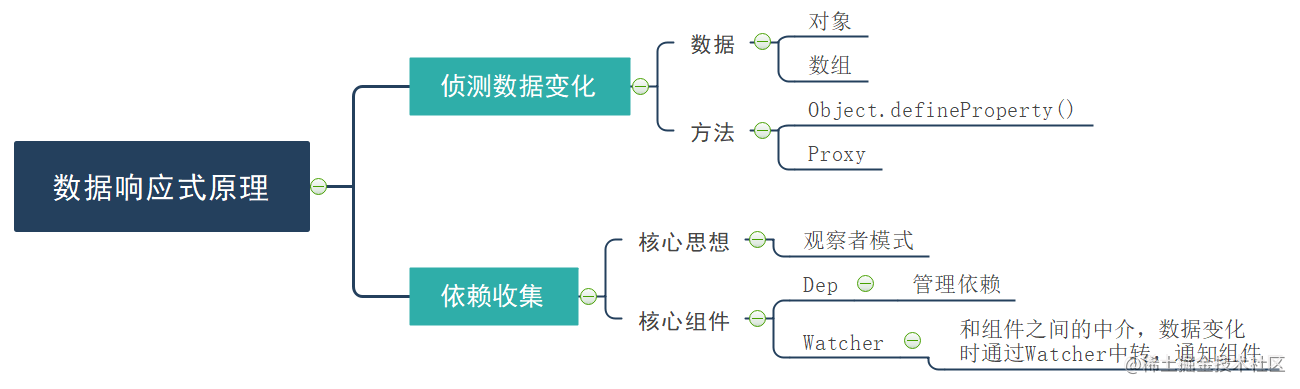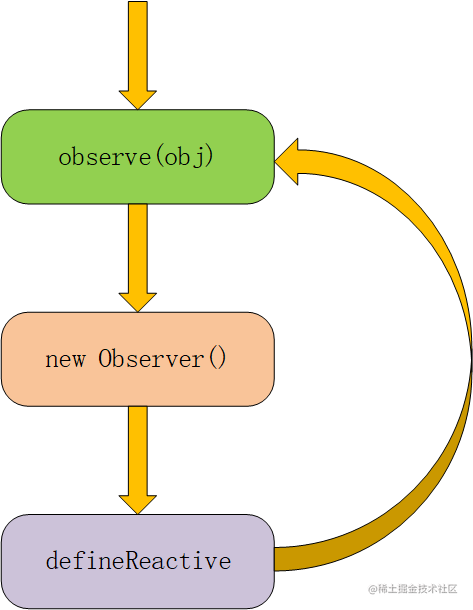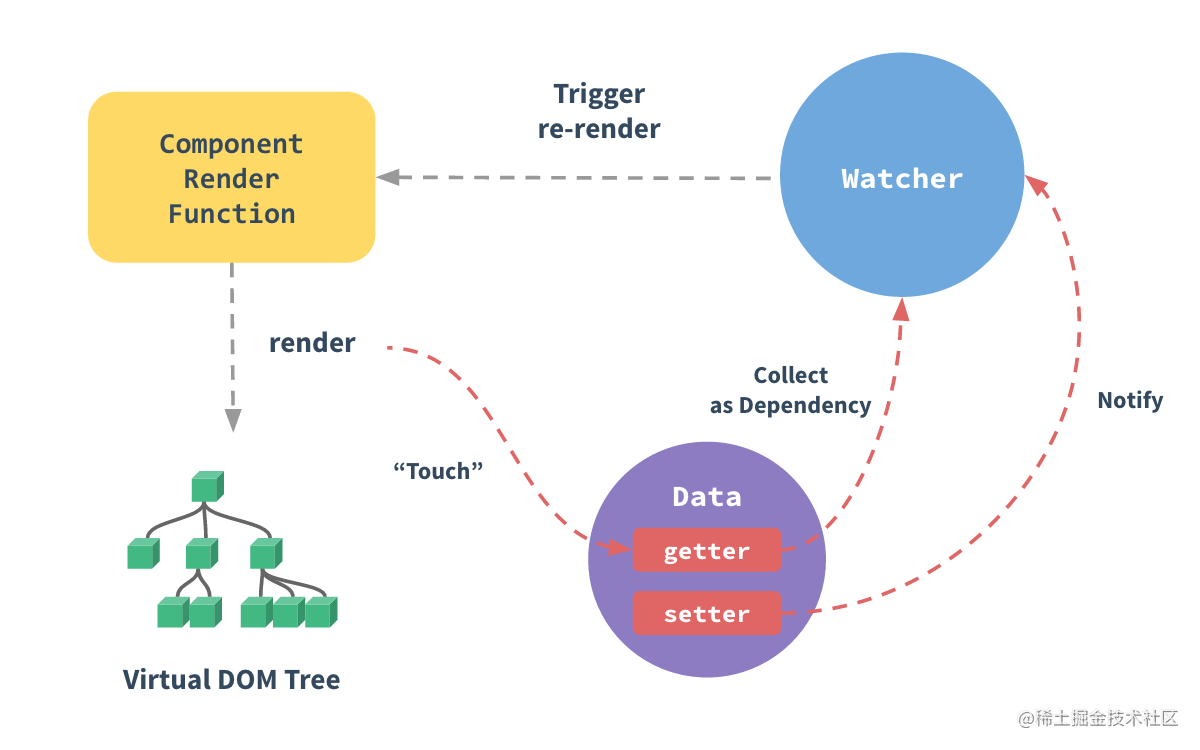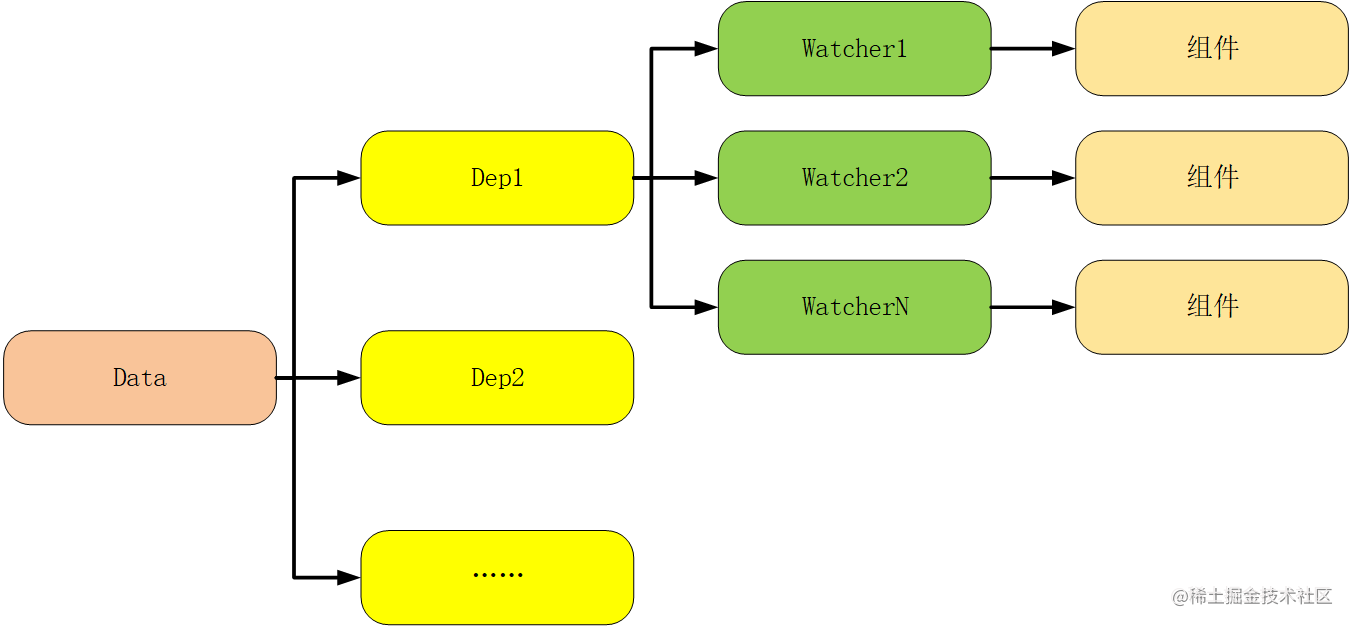## 一、侦测数据变化

### 1.1 非数组对象

``````const obj = {
a: {
m: {
n: 5
}
},
b: 10
};
``````### 1.1.1 observe

``````import Observer from './Observer';

export default function (value) {
// 如果value不是对象，什么都不做(表示该递归到的是基本类型，其变化可被帧听的）
if (typeof value !== 'object') {
return;
}

// Observer实例
let ob;
// __ob__是value上的属性，其值就是对应的Observer实例（表示其已经是可帧听的状态）
if (typeof value.__ob__ !== 'undefined') {
ob = value.__ob__;
}
else {
// 是对象且该上属性还是未能够帧听状态的
ob = new Observer(value);
}

return ob;
}
``````

### 1.1.2 Observer

``````export default class Observer {
constructor(value) {
// 给实例添加__ob__属性
def(value, '__ob__', this, false);
// 检查是数组还是对象
if (!Array.isArray(value)) {
// 若为对象，则进行遍历，将其上的属性变为响应式的
this.walk(value);
}
}

// 对于对象上的属性进行遍历，将其变为响应式的
walk(value) {
for (let key in value) {
defineReactive(value, key);
}
}
}
``````

### 1.1.3 defineReactive

``````export default function defineReactive(data, key, val) {
if (arguments.length === 2) {
val = data[key];
}

// 子元素要进行observe，至此形成了递归
let childOb = observe(val);

Object.defineProperty(data, key, {
// 可枚举
enumerable: true,
// 可配置
configurable: true,
// getter
get() {
console.log(`访问\${key}属性`);
return val;
},
// setter
set(newValue) {
console.log(`改变\${key}的属性为\${newValue}`);
if (val === newValue) {
return;
}
val = newValue;
// 当设置了新值，这个新值也要被observe
childOb = observe(newValue);
}
});
}
``````

### 1.2 数组

Object.defineProperty不能直接监听数组内部的变化，那么数组内容变化应该怎么操作呢？Vue主要采用的是改装数组方法的方式（push、pop、shift、unshift、splice、sort、reverse），在保留其原有功能的前提下，将其新添加的项变为响应式的。

``````// array.js文件
// 得到Array的原型
const arrayPrototype = Array.prototype;

// 以Array.prototype为原型创建arrayMethods对象，并暴露
export const arrayMethods = Object.create(arrayPrototype);

// 要被改写的7个数组方法
const methodsNeedChange = [
'push',
'pop',
'shift',
'unshift',
'splice',
'sort',
'reverse'
];

methodsNeedChange.forEach(methodName => {
//备份原来的方法
const original = arrayMethods[methodName];
// 定义新的方法
def(arrayMethods, methodName, function () {
// 恢复原来的功能
const result = original.apply(this, arguments);

// 将类数组对象转换为数组
const args = [...arguments];
// 数组不会是最外层，所以其上已经添加了Observer实例
const ob = this.__ob__;

// push/unshift/splice会插入新项，需要将插入的新项变成observe的
let inserted = [];

switch (methodName) {
case 'push':
case 'unshift': {
inserted = args;
break;
}
case 'splice': {
inserted = args.slice(2);
break;
}
}

// 对于有插入项的，让新项变为响应的
if (inserted.length) {
ob.observeArray(inserted);
}

ob.dep.notify();

return result;
}, false);
});
``````

``````export default class Observer {
constructor(value) {
// 给实例添加__ob__属性
def(value, '__ob__', this, false);
// 检查是数组还是对象
if (Array.isArray(value)) {
// 改变数组的原型为新改装的内容
Object.setPrototypeOf(value, arrayMethods);
// 让这个数组变为observe
this.observeArray(value);
}
else {
// 若为对象，则进行遍历，将其上的属性变为响应式的
this.walk(value);
}
}

// 对于对象上的属性进行遍历，将其变为响应式的
walk(value) {
for (let key in value) {
defineReactive(value, key);
}
}

// 数组的特殊遍历
observeArray(arr) {
for (let i = 0, l = arr.length; i < l; i++) {
// 逐项进行observe
observe(arr[i]);
}
}
}
``````

## 二、依赖收集1. getter中（Object.defineProperty中的get属性）进行收集依赖后的状态2. 紧接着就是触发依赖，该过程是在setter中进行，当触发依赖时所存储在Dep中的所有Watcher均会被通知并执行，通知其关联的组件更新，例如数据更新的位置是与Dep1所关联的数据，则其上的Watcher1、Watcher2、WatcherN均会被通知并执行。

### 2.1 Dep类

Dep类用于管理依赖，包含依赖的添加、删除、发送消息，是一个典型的观察者模式。

``````export default class Dep {
constructor() {
console.log('DEP构造器');
// 数组存储自己的订阅者，这是Watcher实例
this.subs = [];
}

// 添加订阅
this.subs.push(sub);
}

// 添加依赖
depend() {
// Dep.target指定的全局的位置
if (Dep.target) {
}
}

// 通知更新
notify() {
const subs = this.subs.slice();
for (let i = 0, l = subs.length; i < l; i++) {
subs[i].update();
}
}
}
``````

### 2.2 Watcher类

Watcher类的实例就是依赖，在其实例化阶段会作为依赖存储到Dep中，在对应的数据改变时会更新与该数据相关的Watcher实例，进行对应任务的执行，更新对应组件。

``````export default class Watcher {
constructor(target, expression, callback) {
console.log('Watcher构造器');
this.target = target;
this.getter = parsePath(expression);
this.callback = callback;
this.value = this.get();
}

update() {
this.run();
}

get() {
// 进入依赖收集阶段，让全局的Dep.target设置为Watcher本身，就进入依赖收集阶段
Dep.target = this;
const obj = this.target;
let value;

try {
value = this.getter(obj);
}
finally {
Dep.target = null;
}

return value;
}

run() {
this.getAndInvoke(this.callback);
}

getAndInvoke(cb) {
const value = this.get();

if (value !== this.value || typeof value === 'object') {
const oldValue = this.value;
this.value = value;
cb.call(this.target, value, oldValue);
}
}
}

function parsePath(str) {
const segments = str.split('.');

return obj =>{
for (let i = 0; i < segments.length; i++) {
if (!obj) {
return;
}
obj = obj[segments[i]];
}

return obj;
};
}
``````

### 2.3 defineReactive函数中的set和get函数

Object.defineProperty中的getter阶段进行收集依赖，setter阶段触发依赖。

``````export default function defineReactive(data, key, val) {
const dep = new Dep();
if (arguments.length === 2) {
val = data[key];
}

// 子元素要进行observe，至此形成了递归
let childOb = observe(val);

Object.defineProperty(data, key, {
// 可枚举
enumerable: true,
// 可配置
configurable: true,
// getter
get() {
console.log(`访问\${key}属性`);
// 如果现在处于依赖收集阶段
if (Dep.target) {
dep.depend();
// 其子元素存在的时候也要进行依赖收集(个人认为主要是针对数组）
if (childOb) {
childOb.dep.depend();
}
}
return val;
},
// setter
set(newValue) {
console.log(`改变\${key}的属性为\${newValue}`);
if (val === newValue) {
return;
}
val = newValue;
// 当设置了新值，这个新值也要被observe
childOb = observe(newValue);
// 发布订阅模式，通知更新
dep.notify();
}
});
}
``````

## 参考文献

1.如果觉得这篇文章还不错，来个分享、点赞吧，让更多的人也看到

2.关注公众号执鸢者，领取学习资料（前端“多兵种”资料），定期为你推送原创深度好文